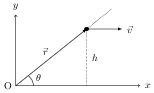# Conservation of Angular Momentum

## Problems from IIT JEE

Problem (IIT JEE 2003): A particle undergoes uniform circular motion. About which point on the plane of the circle, will the angular momentum of the particle remain conserved?

1. centre of the circle.
2. on the circumference of the circle.
3. inside the circle.
4. outside the circle.

Solution: In uniform circular motion, the force on the particle passes through centre of the circle so its torque about this point is zero and angular momentum remains conserved.

Problem (IIT JEE 1997): A mass $m$ is moving with a constant velocity along a line parallel to the $x$-axis, away from the origin. Its angular momentum with respect to the origin,

1. is zero
2. remains constant
3. goes on increasing
4. goes on decreasing

Solution:The angular momentum of the particle about the origin O is given by, \begin{align} \vec{L}&=m\vec{r}\times\vec{v}\nonumber\\ &=m(r\cos\theta\,\hat\imath+r\sin\theta\,\hat\jmath)\times (v\,\hat\imath)\nonumber\\ &=-mvr\sin\theta\,\hat{k}=-mvh\,\hat{k}.\nonumber \end{align} Since $v$ and $h$ are constant, $\vec{L}$ remains constant.